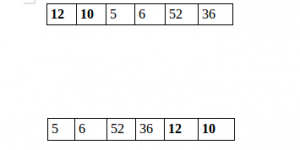Related Articles

# Python Program to Split the array and add the first part to the end

• Difficulty Level : Medium
• Last Updated : 26 Apr, 2020

There is a given an array and split it from a specified position, and move the first part of array add to the end.Examples:

```Input : arr[] = {12, 10, 5, 6, 52, 36}
k = 2
Output : arr[] = {5, 6, 52, 36, 12, 10}
Explanation : Split from index 2 and first
part {12, 10} add to the end .

Input : arr[] = {3, 1, 2}
k = 1
Output : arr[] = {1, 2, 3}
Explanation : Split from index 1 and first
```

## Recommended: Please try your approach on {IDE} first, before moving on to the solution.

 `# Python program to split array and move first``# part to end.`` ` `def` `splitArr(arr, n, k): ``    ``for` `i ``in` `range``(``0``, k): ``        ``x ``=` `arr[``0``]``        ``for` `j ``in` `range``(``0``, n``-``1``):``            ``arr[j] ``=` `arr[j ``+` `1``]``         ` `        ``arr[n``-``1``] ``=` `x``         ` ` ` `# main``arr ``=` `[``12``, ``10``, ``5``, ``6``, ``52``, ``36``]``n ``=` `len``(arr)``position ``=` `2`` ` `splitArr(arr, n, position)`` ` `for` `i ``in` `range``(``0``, n): ``    ``print``(arr[i], end ``=` `' '``)`` ` `# Code Contributed by Mohit Gupta_OMG <(0_o)>   `
Output:
```5 6 52 36 12 10
```

Please refer complete article on Split the array and add the first part to the end for more details!

Another Solution :

 `# Python program to split array and move first``# part to end.`` ` `def` `splitArr(a, n, k): ``   ``b ``=` `a[:k]``   ``return` `(a[k::]``+``b[::])``         ` ` ` `# main``arr ``=` `[``12``, ``10``, ``5``, ``6``, ``52``, ``36``]``n ``=` `len``(arr)``position ``=` `2``arr ``=` `splitArr(arr, n, position)``for` `i ``in` `range``(``0``, n): ``    ``print``(arr[i], end ``=` `' '``)`
Output:
```5 6 52 36 12 10
```

My Personal Notes arrow_drop_up